﻿ Example 2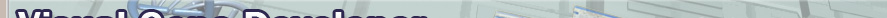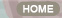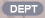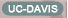Home > Tutorial > 6.Module development > Example 2

Module development

Example 2:

Calculate Gibbs energy of mRNA secondary structure and then screen out a given gene construct if calculated Gibbs energy is lower than the threshold value. It is a predefined script named as 'Check stable mRNA structure'

The module retrieves sequence information ('Target Gene Component ID') using GeneConstruct class and then generates lots of sequence segments specified by 'Window size'. The moving window scans along the sequence with a given 'Step size'. The Visual Gene Developer predicts mRNA secondary structure and calculates Gibb's free energy for each segment. If Gibb's energy is lower than a specified value ('Threshold value'), it returns 'Not pass' which means the gene includes stable mRNA secondary structure. If all sequence segments of the target gene are more unstable than the expected value ('Threshold value'), the module returns 'Pass'.

Step 1. Develop a new PropertyBag. Check a predefined 'mRNA Gibbs Energy Check' as shown in the figure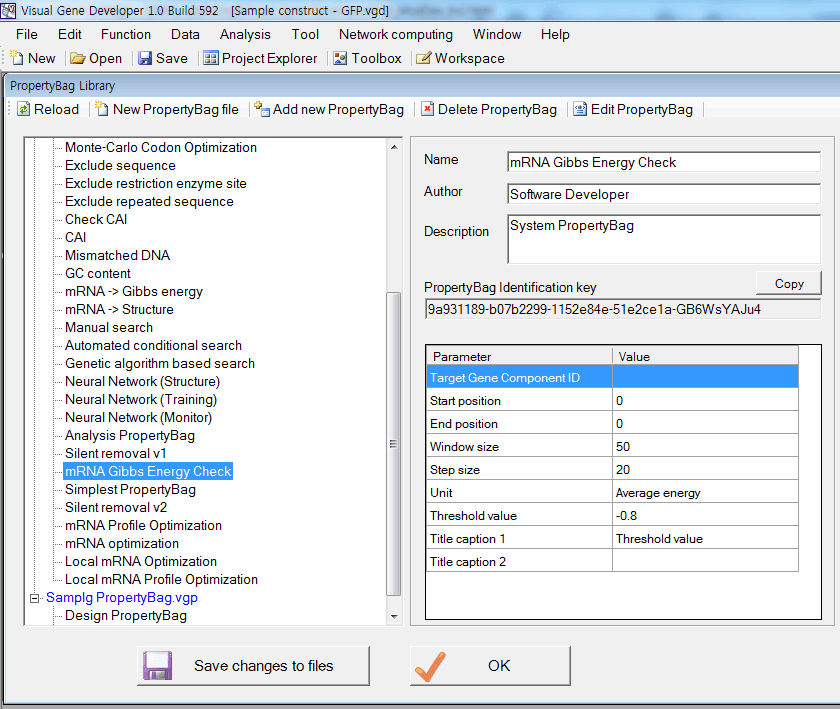Because a user can't edit the PropertyBag, we show detailed setting in the below

The 1st parameter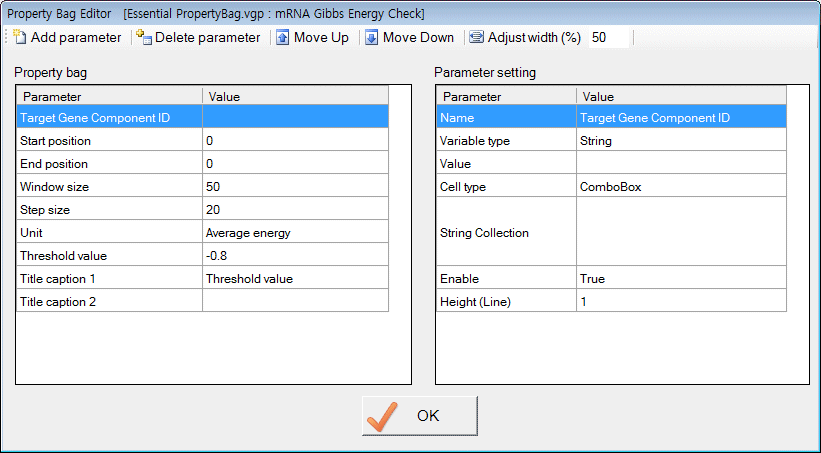The 2nd parameter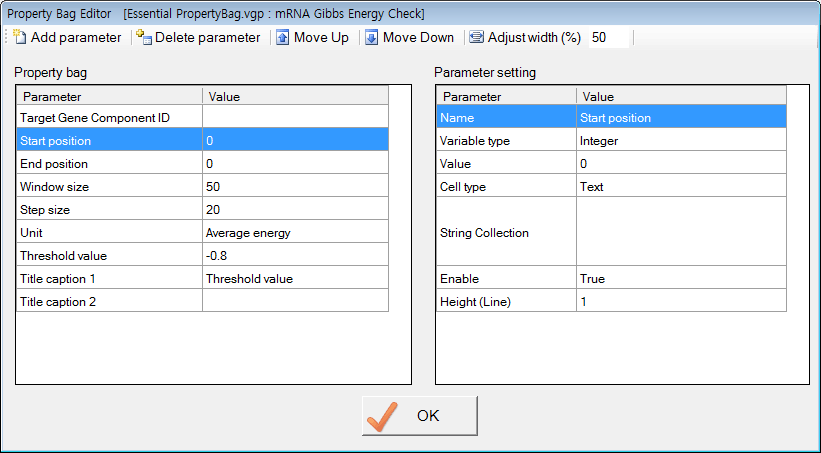The 3rd parameter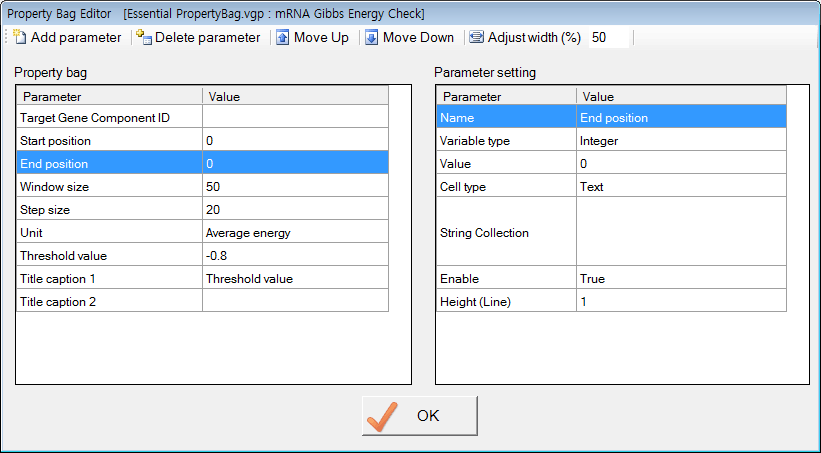The 4th parameterThe 5th parameter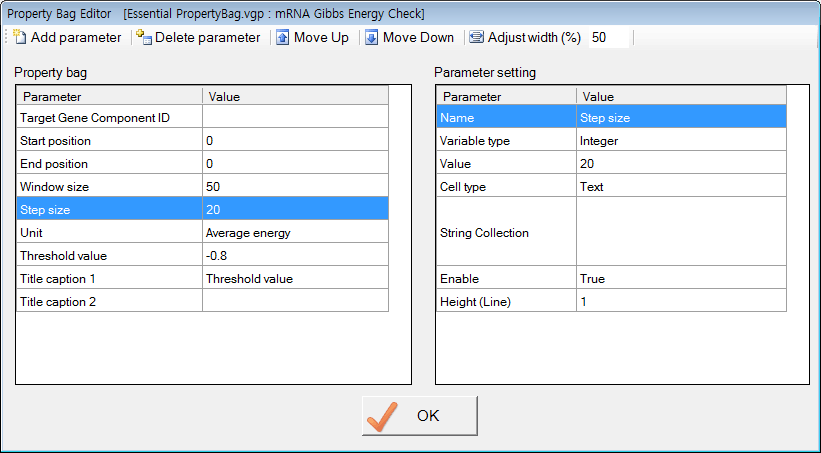The 6th parameter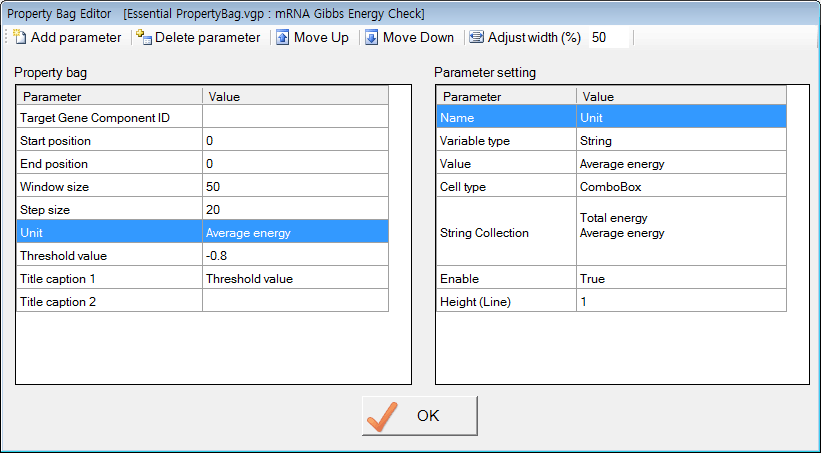The 7th parameter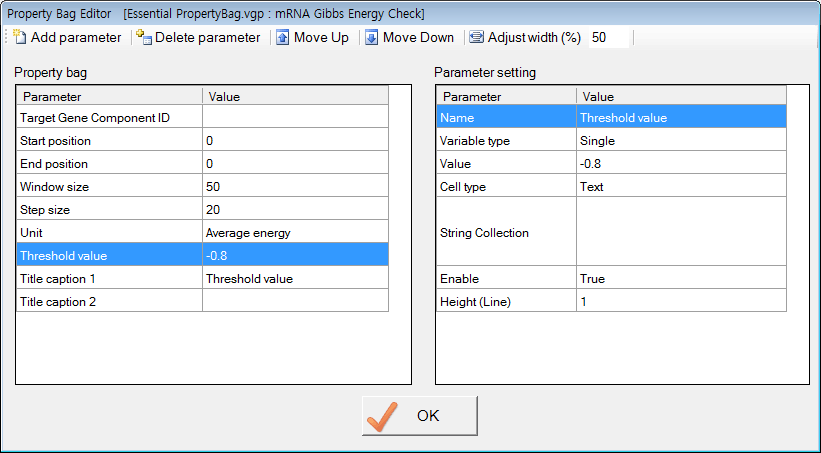The 8th parameter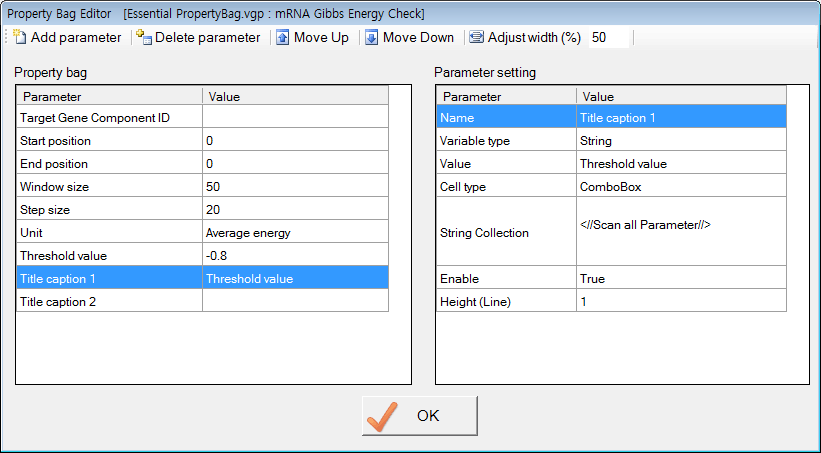Step 2. Develop source code.

1. Generate new function

2. Check on the 'Registration' and set Category to be 'Constraint'

3. Don't forget attaching a PropertyBag.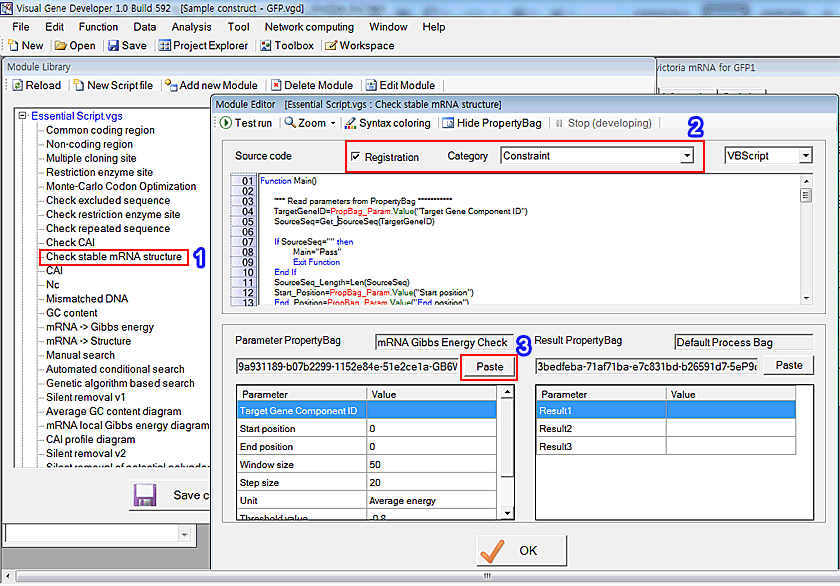VBScript source code

 Function Main()      '*** Read parameters from PropertyBag ***********      TargetGeneID=PropBag_Param.Value("Target Gene Component ID")      SourceSeq=Get_SourceSeq(TargetGeneID)      If SourceSeq="" then           Main="Pass"           Exit Function      End If      SourceSeq_Length=Len(SourceSeq)      Start_Position=PropBag_Param.Value("Start position")      End_Position=PropBag_Param.Value("End position")      Window_Size=PropBag_Param.Value("Window size")      Step_Size=PropBag_Param.Value("Step size")      Unit=PropBag_Param.Value("Unit")      Threshold_Value=PropBag_Param.Value("Threshold value")        '*** Check parameters ***********      If Start_Position<=0 then Start_Position=1      If End_Position >SourceSeq_Length then End_Position=SourceSeq_Length      If Start_Position>End_Position then           TempValue=Start_Position           Start_Position=End_Position           End_Position=TempValue      End If      If Window_Size>100 then           Window_Size=100           PropBag_Param.Value("Window size")="100"      End If      If Window_Size<10 then           Window_Size=10           PropBag_Param.Value("Window size")="10"      End If      If Step_Size>100 then           Step_Size=100           PropBag_Param.Value("Step size")="100"      End If      If Step_Size<5 then           Step_Size=5           PropBag_Param.Value("Step size")="5"      End If      '*** Check mRNA Gibbs free energy ************************************************      For Current_Position=Start_Position to End_Position - Step_Size step Step_Size    '<----- Moving window           AppService.InstantMsg "Analyzing mRNA structure at " + CStr(Current_Position)           TestSeq=Mid(SourceSeq,Current_Position,Window_Size)      '<---- Generates fragment of target gene           TestSeq_Length=Len(TestSeq)           Call mRNApredict.Calculate(TestSeq,True)           GibbsEnergy=mRNApredict.GibbsFreeEnergy ()           If Unit="Total energy" then                If -GibbsEnergy > -Threshold_Value then                     Main= "Not pass"                     Exit Function                End If           Else                If (-GibbsEnergy/TestSeq_Length) > -Threshold_Value then                     Main="Not pass"                     Exit Function                End If           End If      Next      Main="Pass" End Function Function Get_SourceSeq(TargetGeneName)       '<---- Get target sequence. Use this module as a template for module development      TargetGeneConstructIndex=GeneConstruct.CurrentConstructIndex      TargetGeneIndex=GeneConstruct.ComponentIndex(TargetGeneConstructIndex,TargetGeneName)      Get_SourceSeq=GeneConstruct.ParameterValue(TargetGeneConstructIndex,TargetGeneIndex,"Modified DNA") End Function